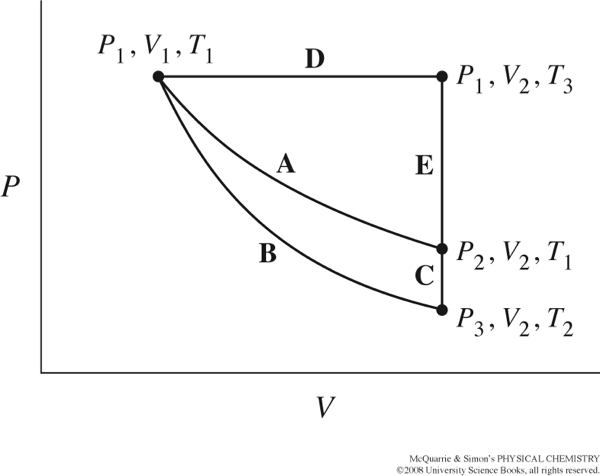Groupwork 5 Adiabatic Cooling

Name: ______________________________

Section: _____________________________

Student ID#:__________________________

Work in groups on these problems. You should try to answer the questions without referring to your textbook. If you get stuck, try asking another group for help.

The figure below from your text, shows several different paths that could be taken as thermodynamic variables, P, T, and V, change.Path A represents a reversible isothermal expansion/compression.

Path B represents a reversible adiabatic expansion/compression.

Path C completes the cycle.

Consider this diagram for an ideal gas following $$PV=nRT$$. Rank the pressure values for $$P_1,P_2,P_3$$ from largest to smallest. What is larger, $$V_1$$ or $$V_2$$?

What is larger, $$T_1$$ or $$T_2$$? Justify your answer.

For Path A, write down an expression describing dUA.

For Path B, write down an expression describing dUB.

For Path C, write down an expression describing dUC.

What is dU for the whole cycle, that is Path A to Path B to Path C?

What does this tell you about dUB and dUC?

Write down mathematical expressions for dqC and dwB. How are these two expressions related? If you want to integrate dqC and dwB, how can you simplify the expression so that you have only one variable on each side of the equation?

How are the change in volume and change in temperature related to each other?